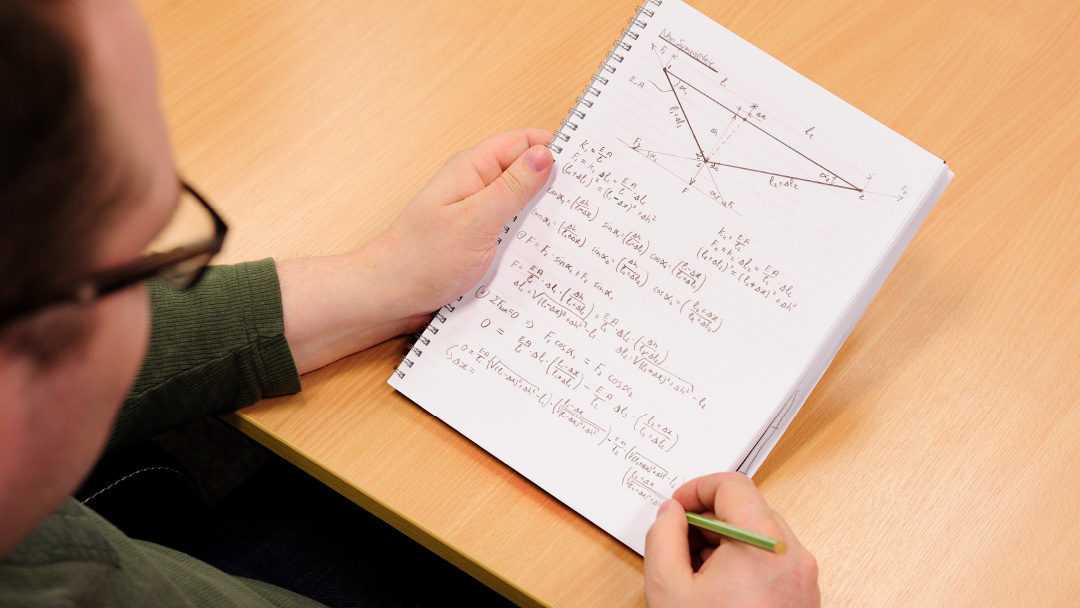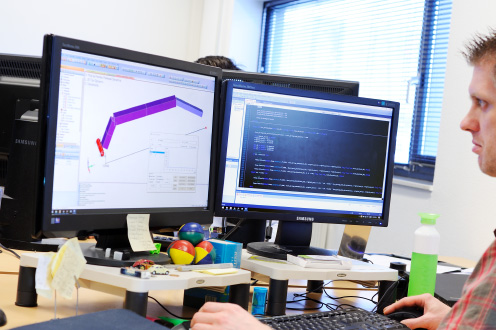# LINEARCALCULATIONS

Linear structural analysis is by far the most cost-effective method within the Finite Element Method’s range. Especially steel and aluminium structures are well suited for analysis with this method. Complex shapes and huge structures can be analysed faster and with a higher degree of accuracy than by analysing them by hand. Large numbers of load-cases can be assessed without the need for time consuming calculations.

### Linearity assumptions

In analysing designs for strength and deformation, most methods require the material behaviour to be linear in material (stiffness and other material properties do not change with the speed of loading or load magnitude) and geometry (deformations need to be small, so that load directions do not need to be updated with this deformation). Femtec is able to work within these constraints and use the powerful and fast linear calculations to our customer’s advantage. Especially in the early stages of a design, fast linear calculations will benefit the designer in choosing the right concept.

### The power of linear analysis

Within the constraints of linearity, the finite element analysis offers a great advantage over traditional calculations by hand; the geometry complexity can be much greater. In hand calculations, the geometry needs to be reduced to simple shapes (bars, beams, pipes, and plates, et cetera) for which calculation formulae are known. Using the finite element method, the shape complexity and the extent of the design are limited only by the calculation power of the computer used. Femtec has a very powerful calculation server at its disposal, to perform these extensive linear calculations in a timely fashion.### Metal Alloys: the perfect linear material

Steel and aluminium are prime examples of construction materials that have a large range of linear behaviour before their yield point signals the transition into non-linearity. If a load would bring these materials beyond that yield point, the construction made from that material would experience a lasting deformation even after the load is removed. For most constructions, this effect is not desirable and therefore linear analysis is a perfect fit for these materials, as the yield point of the material used often determines allowable stress. Femtec has extensive experience calculating with the great range of different steel and aluminium alloys available.

### Non-static linear analysis

Static analysis suggests that the construction under investigation does not move and that moving constructions need a different more complex method of analysis. Fortunately, this is not completely true; using D’Alembert’s principle of inertial forces, we are able to calculate inertial forces working on a construction in motion using a pseudo-static load. Load-cases involving acceleration as well as discrete forces and pressures can be analysed using the linear (pseudo-) static method, as long as these forces are perfectly balanced against the inertial forces. Femtec engineers are able to perform this tricky task balancing external loads with inertial forces working on moving structures.

### Significantly reduces the number of load cases

Working within the confines of linear analysis has another great advantage besides the speed of the analysis. The linearity principle gives us the opportunity to scale and combine results from individual load-cases. This means that if several load-cases only change uniformly in the magnitude of the load, the results of each of those can be derived from the results of a single finite element analysis of one of those load-cases. Also, the results of several load-cases can be linearly combined, reducing the number of needed finite element calculations even further if load-cases can be defined as a linear combination of a limited amount of simpler load-cases. Femtec has the tools and experience to find and take advantage of those linear combinations in our customer’s load requirements.

## Linear dynamicsLinear calculations are often the basis of dynamic calculations, for example dynamic behaviour or fatigue assessment. Modal analysis is done with a linear solution method, but, with additional calculations, can predict dynamic behaviour of constructions. More often than not, linear calculations are done and combined for fatigue calculations, which require extensive post-processing of those linear results.

Learn more